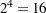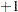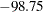Previous Page | Next Page

 The GLM Procedure

## Example 39.11 Analysis of a Screening Design

Yin and Jillie (1987) describe an experiment performed on a nitride etch process for a single wafer plasma etcher. The experiment is run using four factors: cathode power (power), gas flow (flow), reactor chamber pressure (pressure), and electrode gap (gap). Of interest are the main effects and interaction effects of the factors on the nitride etch rate (rate). The following statements create a SAS data set named HalfFraction, containing the factor settings and the observed etch rate for each of eight experimental runs.

```data HalfFraction;
input power flow pressure gap rate;
datalines;
0.8   4.5 125 275     550
0.8   4.5 200 325     650
0.8 550.0 125 325     642
0.8 550.0 200 275     601
1.2   4.5 125 325     749
1.2   4.5 200 275    1052
1.2 550.0 125 275    1075
1.2 550.0 200 325     729
;
```

Notice that each of the factors has just two values. This is a common experimental design when the intent is to screen from the many factors that might affect the response the few that actually do. Since there aredifferent possible settings of four two-level factors, this design with only eight runs is called a "half fraction." The eight runs are chosen specifically to provide unambiguous information on main effects at the cost of confounding interaction effects with each other.

One way to analyze these data is simply to use PROC GLM to compute an analysis of variance, including both main effects and interactions in the model. The following statements demonstrate this approach.

```proc glm data=HalfFraction;
class power flow pressure gap;
model rate=power|flow|pressure|gap@2;
run;
```

The "@2" notation in the MODEL statement includes all main effects and two-factor interactions between the factors. The output is shown in Output 39.11.1.

Output 39.11.1 Analysis of Variance for Nitride Etch Process Half Fraction
The GLM Procedure

Class Level Information
Class Levels Values
power 2 0.8 1.2
flow 2 4.5 550
pressure 2 125 200
gap 2 275 325

 Number of Observations Read 8 8

The GLM Procedure

Dependent Variable: rate

Source DF Sum of Squares Mean Square F Value Pr > F
Model 7 280848.0000 40121.1429 . .
Error 0 0.0000 .
Corrected Total 7 280848.0000

R-Square Coeff Var Root MSE rate Mean
1.000000 . . 756.0000

Source DF Type I SS Mean Square F Value Pr > F
power 1 168780.5000 168780.5000 . .
flow 1 264.5000 264.5000 . .
power*flow 1 200.0000 200.0000 . .
pressure 1 32.0000 32.0000 . .
power*pressure 1 1300.5000 1300.5000 . .
flow*pressure 1 78012.5000 78012.5000 . .
gap 1 32258.0000 32258.0000 . .
power*gap 0 0.0000 . . .
flow*gap 0 0.0000 . . .
pressure*gap 0 0.0000 . . .

Source DF Type III SS Mean Square F Value Pr > F
power 1 168780.5000 168780.5000 . .
flow 1 264.5000 264.5000 . .
power*flow 0 0.0000 . . .
pressure 1 32.0000 32.0000 . .
power*pressure 0 0.0000 . . .
flow*pressure 0 0.0000 . . .
gap 1 32258.0000 32258.0000 . .
power*gap 0 0.0000 . . .
flow*gap 0 0.0000 . . .
pressure*gap 0 0.0000 . . .

Notice that there are no error degrees of freedom. This is because there are 10 effects in the model (4 main effects plus 6 interactions) but only 8 observations in the data set. This is another cost of using a fractional design: not only is it impossible to estimate all the main effects and interactions, but there is also no information left to estimate the underlying error rate in order to measure the significance of the effects that are estimable.

Another thing to notice in Output 39.11.1 is the difference between the Type I and Type III ANOVA tables. The rows corresponding to main effects in each are the same, but no Type III interaction tests are estimable, while some Type I interaction tests are estimable. This indicates that there is aliasing in the design: some interactions are completely confounded with each other.

In order to analyze this confounding, you should examine the aliasing structure of the design by using the ALIASING option in the MODEL statement. Before doing so, however, it is advisable to code the design, replacing low and high levels of each factor with the valuesand, respectively. This puts each factor on an equal footing in the model and makes the aliasing structure much more interpretable. The following statements code the data, creating a new data set named Coded.

```data Coded; set HalfFraction;
power    = -1*(power   =0.80) + 1*(power   =1.20);
flow     = -1*(flow    =4.50) + 1*(flow    =550 );
pressure = -1*(pressure=125 ) + 1*(pressure=200 );
gap      = -1*(gap     =275 ) + 1*(gap     =325 );
run;
```

The following statements use the GLM procedure to reanalyze the coded design, displaying the parameter estimates as well as the functions of the parameters that they each estimate.

```proc glm data=Coded;
model rate=power|flow|pressure|gap@2 / solution aliasing;
run;
```

The parameter estimates table is shown in Output 39.11.2.

Output 39.11.2 Parameter Estimates and Aliases for Nitride Etch Process Half Fraction
The GLM Procedure

Dependent Variable: rate

Parameter Estimate   Standard Error t Value Pr > |t| Expected Value
Intercept 756.0000000   . . . Intercept
power 145.2500000   . . . power
flow 5.7500000   . . . flow
power*flow -5.0000000 B . . . power*flow + pressure*gap
pressure 2.0000000   . . . pressure
power*pressure -12.7500000 B . . . power*pressure + flow*gap
flow*pressure -98.7500000 B . . . flow*pressure + power*gap
gap -63.5000000   . . . gap
power*gap 0.0000000 B . . .
flow*gap 0.0000000 B . . .
pressure*gap 0.0000000 B . . .

In the "Expected Value" column, notice that, while each of the main effects is unambiguously estimated by its associated term in the model, the expected values of the interaction estimates are more complicated. For example, the relatively large effect () corresponding to flow*pressure actually estimates the combined effect of flow*pressure and power*gap. Without further information, it is impossible to disentangle these aliased interactions; however, since the main effects of both power and gap are large and those for flow and pressure are small, it is reasonable to suspect that power*gap is the more "active" of the two interactions.

Fortunately, eight more runs are available for this experiment (the other half fraction). The following statements create a data set containing these extra runs and add it to the previous eight, resulting in a fullrun replicate. Then PROC GLM displays the analysis of variance again.

```data OtherHalf;
input power flow pressure gap rate;
datalines;
0.8   4.5 125 325     669
0.8   4.5 200 275     604
0.8 550.0 125 275     633
0.8 550.0 200 325     635
1.2   4.5 125 275    1037
1.2   4.5 200 325     868
1.2 550.0 125 325     860
1.2 550.0 200 275    1063
;
data FullRep;
set HalfFraction OtherHalf;
run;
```
```proc glm data=FullRep;
class power flow pressure gap;
model rate=power|flow|pressure|gap@2;
run;
```

The results are displayed in Output 39.11.3.

Output 39.11.3 Analysis of Variance for Nitride Etch Process Full Replicate
The GLM Procedure

Class Level Information
Class Levels Values
power 2 0.8 1.2
flow 2 4.5 550
pressure 2 125 200
gap 2 275 325

 Number of Observations Read 16 16

The GLM Procedure

Dependent Variable: rate

Source DF Sum of Squares Mean Square F Value Pr > F
Model 10 521234.1250 52123.4125 25.58 0.0011
Error 5 10186.8125 2037.3625
Corrected Total 15 531420.9375

R-Square Coeff Var Root MSE rate Mean
0.980831 5.816175 45.13715 776.0625

Source DF Type I SS Mean Square F Value Pr > F
power 1 374850.0625 374850.0625 183.99 <.0001
flow 1 217.5625 217.5625 0.11 0.7571
power*flow 1 18.0625 18.0625 0.01 0.9286
pressure 1 10.5625 10.5625 0.01 0.9454
power*pressure 1 1.5625 1.5625 0.00 0.9790
flow*pressure 1 7700.0625 7700.0625 3.78 0.1095
gap 1 41310.5625 41310.5625 20.28 0.0064
power*gap 1 94402.5625 94402.5625 46.34 0.0010
flow*gap 1 2475.0625 2475.0625 1.21 0.3206
pressure*gap 1 248.0625 248.0625 0.12 0.7414

Source DF Type III SS Mean Square F Value Pr > F
power 1 374850.0625 374850.0625 183.99 <.0001
flow 1 217.5625 217.5625 0.11 0.7571
power*flow 1 18.0625 18.0625 0.01 0.9286
pressure 1 10.5625 10.5625 0.01 0.9454
power*pressure 1 1.5625 1.5625 0.00 0.9790
flow*pressure 1 7700.0625 7700.0625 3.78 0.1095
gap 1 41310.5625 41310.5625 20.28 0.0064
power*gap 1 94402.5625 94402.5625 46.34 0.0010
flow*gap 1 2475.0625 2475.0625 1.21 0.3206
pressure*gap 1 248.0625 248.0625 0.12 0.7414

With 16 runs, the analysis of variance tells the whole story: all effects are estimable and there are five degrees of freedom left over to estimate the underlying error. The main effects of power and gap and their interaction are all significant, and no other effects are. Notice that the Type I and Type III ANOVA tables are the same; this is because the design is orthogonal and all effects are estimable.

This example illustrates the use of the GLM procedure for the model analysis of a screening experiment. Typically, there is much more involved in performing an experiment of this type, from selecting the design points to be studied to graphically assessing significant effects, optimizing the final model, and performing subsequent experimentation. Specialized tools for this are available in SAS/QC software, in particular the ADX Interface and the FACTEX and OPTEX procedures. See SAS/QC User’s Guide for more information.Previous Page | Next Page | Top of Page